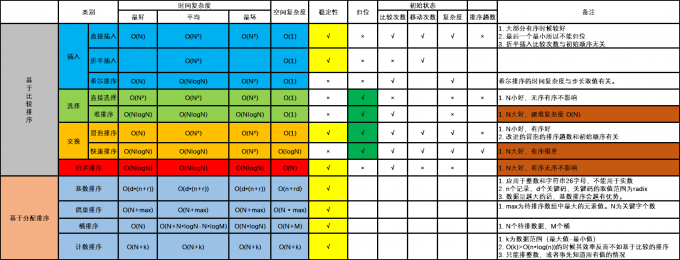# 《数据结构与算法》第10章 排序(C语言)

## 10.1排序分类

1、稳定排序和非稳定排序

2、内排序和外排序

1） 比较两个关键字的大小 ；

2）将记录从一个位置移动到另一个位置。## 10.2插入排序

### 10.2.1直接插入排序

#define maxsize 1024 //文件最大长度
typedef int key_t;  //设key为整数
typedef struct //记录类型
{
key_t key; //记录关键字
.....  //记录其他域
}Retype;
typedef struct //文件或表的类型
{
Retype R[maxsize + 1]; //文件存储空间
int len; //当前记录数
}sqfile;  

void Insort(sqfile F)
{
int i,j;
for(i = 2;i <= F;i++) //插入n - 1个记录
{
F.R = F.R[i]; //带插入记录先存于监视哨
j = i - 1;
while(F.R.key < F.R[j].key) //key 比较
{
F.R[j + 1] = F[j]; //记录顺序后移
j--;
}
F.R[j + 1] = F.R; //原R[i]插入j + 1位置
}
}  

### 10.2.2折半插入排序

void Binsort(sqfile F) //对文件F折半插入排序的算法
{
int i,j,high,low,mid;
for(i = 2;i <= F.len,i++) //插入n-1个记录
{
F.R = F.R[i];//待插入记录存入监视哨
low = 1;
high = i - 1;
while(low < high) //查找 R[i]的位置，即low = high 时；
{
mid = (low + high)/2;
if(F.R.key >= F.R[mid].key)
low = mid + 1; //调整下界
else
high =mid - 1;//调整上界
}

for(j = i - 1;j >= low;j--)
F.R[j + 1] = F.R[j]; //记录瞬移

F.R[low] = F.R; //原R[i]插入low位置
}
}  

## 10.3交换排序

### 10.3.1冒泡排序

k1~k2，若 k1 > k2 ，则R1 与 R2 交换；
…..
k n-1 ~ kn，若kn-1 > kn ，则Rn-1 与 Rn 交换。

void Bubsort(sqfile F)
{
int i,flag; //flag 作为记录交换的标志
Retype temp;
for(i = F.len;i > 2;i--) //最多n - 1次排序
{
flag = 0;
for(j = 1; j <= i - 1;j++) //一趟起泡排序
{
if(F.R[j].key > F.R[j + 1].key) //两两比较
{
temp = F.R[j]; //交换
F.R[j] = F.R[j + 1];
F.R[j + 1] = temp;
flag = 1;
}
flag = 1;
}
if(flag == 0) //无记录交换时排序完毕
break;
}
}  

### 10.3.2快速排序

1）设置两个变量i、j，排序开始的时候：i=0，j=N-1；
2）以第一个数组元素作为关键数据，赋值给key，即key=A；
3）从j开始向前搜索，即由后开始向前搜索(j–)，找到第一个小于key的值A[j]，将A[j]和A[i]互换；
4）从i开始向后搜索，即由前开始向后搜索(i++)，找到第一个大于key的A[i]，将A[i]和A[j]互换；
5）重复第3、4步，直到i=j； (3,4步中，没找到符合条件的值，即3中A[j]不小于key,4中A[i]不大于key的时候改变j、i的值，使得j=j-1，i=i+1，直至找到为止。找到符合条件的值，进行交换的时候i， j指针位置不变。另外，i==j这一过程一定正好是i+或j-完成的时候，此时令循环结束）。

1）一趟快排算法

int Partition(sqfile *L,int low ,int high)
{
key_t pivotkey; //基准key
L->r = L->r[low]; //存入基准记录
pivotkey = L->r[low].key; //存入基准key

while(low < high)
{
while(low < high && L->r[high].key >= pivotkey) //逆序比较
high--;
if(low < high)
L->r[low] = L->r[high]; //比 pivotkey小的key左移

while(low < high && L->r[low].key <= pivotkey) //正序比较
low++;
if(low < high)
L->r[high] = L->r[low]; //比 pivotkey 大的右移
}
L->r[low] = L->r; //基准记录存入第i位置

return low; //返回基准位置
}  

2）对文件L快速排序的算法（递归）

void QSort(sqfile *L,int low ,int high)
{
int  pivotloc;
int i;

if(low < high)
{
pivotloc = Partition(L,low,high); //将L分成两部分
QSort(L,low,pivotloc - 1); //对左部再排序
QSort(L,pivotloc + 1,high); //对右部再排序
}
}  

#include <stdio.h>
#define maxsize 1024
typedef int key_t;
typedef struct
{
key_t key;
}Retype;

typedef struct
{
Retype r[maxsize + 1];
int len;
}sqlist;

int Partition(sqlist *L,int low ,int high)
{
key_t pivotkey;
L->r = L->r[low];
pivotkey = L->r[low].key;

while(low < high)
{
while(low < high && L->r[high].key >= pivotkey)
high--;
if(low < high)
L->r[low] = L->r[high];

while(low < high && L->r[low].key <= pivotkey)
low++;
if(low < high)
L->r[high] = L->r[low];
}
L->r[low] = L->r;

return low;
}
void QSort(sqlist *L,int low ,int high)
{
int  pivotloc;
int i;

if(low < high)
{
pivotloc = Partition(L,low,high);
QSort(L,low,pivotloc - 1);
QSort(L,pivotloc + 1,high);
}
}
int main()
{
int i;
sqlist L;
L.len = 8;
key_t a = {50,36,66,76,36,12,25,95};

for(i = 1; i < 9; i++)
{
L.r[i].key = a[i - 1];
}

printf("Begin sorting!\n");

QSort(&L,1,8);
for(i = 1; i < 9; i++)
{
printf("%d ",L.r[i].key);
}
printf("\n");

return 0;
}  

### 资源获取方法

1.关注公众号[嵌入式实验楼]
2.在公众号回复关键词[Data Structures and Algorithms]获取资料提取码